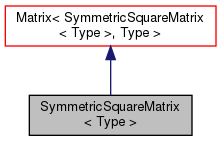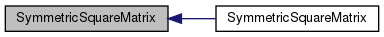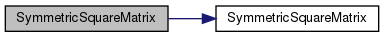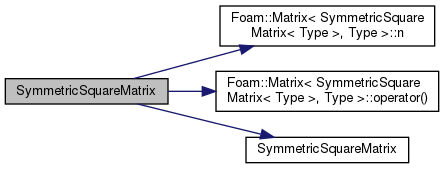The OpenFOAM Foundation
SymmetricSquareMatrix< Type > Class Template Reference

A templated 2D square symmetric matrix of objects of <T>, where the n x n matrix dimension is known and used for subscript bounds checking, etc. More...

Inheritance diagram for SymmetricSquareMatrix< Type >:[legend]
Collaboration diagram for SymmetricSquareMatrix< Type >:[legend]

## Public Member Functions

SymmetricSquareMatrix ()
Null constructor. More...

SymmetricSquareMatrix (const label n)
Construct given number of rows/columns. More...

SymmetricSquareMatrix (const label n, const zero)
Construct given number of rows/columns, initializing to zero. More...

SymmetricSquareMatrix (const label n, const Identity< Type >)
Construct given number of rows/columns,. More...

SymmetricSquareMatrix (const label n, const Type &)
Construct with given number of rows/columns. More...

SymmetricSquareMatrix (Istream &)
Construct from Istream. More...

autoPtr< SymmetricSquareMatrix< Type > > clone () const
Clone. More...Public Member Functions inherited from Matrix< SymmetricSquareMatrix< Type >, Type >
Matrix ()
Null constructor. More...

Matrix (const label m, const label n)
Construct given number of rows and columns. More...

Matrix (const label m, const label n, const zero)
Construct with given number of rows and columns. More...

Matrix (const label m, const label n, const Type &)
Construct with given number of rows and columns. More...

Matrix (const mType &)
Copy constructor. More...

Matrix (const Matrix< Form2, Type > &)
Copy constructor from matrix of a different form. More...

Matrix (const ConstMatrixBlock< MatrixType > &)
Construct from a block of another matrix. More...

Matrix (const MatrixBlock< MatrixType > &)
Construct from a block of another matrix. More...

Matrix (Istream &)
Construct from Istream. More...

autoPtr< mTypeclone () const
Clone. More...

~Matrix ()
Destructor. More...

label m () const
Return the number of rows. More...

label n () const
Return the number of columns. More...

label size () const
Return the number of elements in matrix (m*n) More...

const Type * v () const
Return element vector of the constant Matrix. More...

Type * v ()
Return element vector of the Matrix. More...

ConstMatrixBlock< mTypeblock (const label m, const label n, const label mStart, const label nStart) const

ConstMatrixBlock< mTypeblock (const label mStart, const label nStart) const

MatrixBlock< mTypeblock (const label m, const label n, const label mStart, const label nStart)

MatrixBlock< mTypeblock (const label mStart, const label nStart)

Foam::ConstMatrixBlock< Foam::Matrix< SymmetricSquareMatrix< Type >, Type > > block (const label mStart, const label nStart) const

Foam::MatrixBlock< Foam::Matrix< SymmetricSquareMatrix< Type >, Type > > block (const label mStart, const label nStart)

ConstMatrixBlock< mTypecol (const label m, const label rowStart) const

ConstMatrixBlock< mTypecol (const label m, const label mStart, const label nStart) const

MatrixBlock< mTypecol (const label m, const label rowStart)

MatrixBlock< mTypecol (const label m, const label mStart, const label nStart)

void checki (const label i) const
Check index i is within valid range (0 ... m-1). More...

void checkj (const label j) const
Check index j is within valid range (0 ... n-1). More...

void clear ()
Clear the Matrix, i.e. set sizes to zero. More...

void transfer (mType &)
Transfer the contents of the argument Matrix into this Matrix. More...

void setSize (const label m, const label n)
Resize the matrix preserving the elements. More...

void shallowResize (const label m, const label n)
Resize the matrix without reallocating storage (unsafe) More...

SymmetricSquareMatrix< Type > T () const
Return the transpose of the matrix. More...

Type * operator[] (const label)
Return subscript-checked row of Matrix. More...

const Type * operator[] (const label) const
Return subscript-checked row of constant Matrix. More...

const Type & operator() (const label i, const label j) const
(i, j) const element access operator More...

Type & operator() (const label i, const label j)
(i, j) element access operator More...

void operator= (const mType &)
Assignment operator. Takes linear time. More...

void operator= (const ConstMatrixBlock< MatrixType > &)
Assignment to a block of another matrix. More...

void operator= (const MatrixBlock< MatrixType > &)
Assignment to a block of another matrix. More...

void operator= (const zero)
Assignment of all elements to zero. More...

void operator= (const Type &)
Assignment of all elements to the given value. More...Public Types inherited from Matrix< SymmetricSquareMatrix< Type >, Type >
typedef Matrix< SymmetricSquareMatrix< Type >, Type > mType
Matrix type. More...

typedef Type cmptType
Component type. More...Static Public Member Functions inherited from Matrix< SymmetricSquareMatrix< Type >, Type >
static const mTypenull ()
Return a null Matrix. More...

## Detailed Description

### template<class Type> class Foam::SymmetricSquareMatrix< Type >

A templated 2D square symmetric matrix of objects of <T>, where the n x n matrix dimension is known and used for subscript bounds checking, etc.

Source files

Definition at line 52 of file SymmetricSquareMatrix.H.

## ◆ SymmetricSquareMatrix() [1/6]

 SymmetricSquareMatrix ( )
inline

Null constructor.

Definition at line 29 of file SymmetricSquareMatrixI.H.

Referenced by SymmetricSquareMatrix< Type >::SymmetricSquareMatrix().

Here is the caller graph for this function:## ◆ SymmetricSquareMatrix() [2/6]

 SymmetricSquareMatrix ( const label n )
inline

Construct given number of rows/columns.

Definition at line 36 of file SymmetricSquareMatrixI.H.

Here is the call graph for this function:## ◆ SymmetricSquareMatrix() [3/6]

 SymmetricSquareMatrix ( const label n, const zero )
inline

Construct given number of rows/columns, initializing to zero.

Definition at line 44 of file SymmetricSquareMatrixI.H.

Here is the call graph for this function:## ◆ SymmetricSquareMatrix() [4/6]

 SymmetricSquareMatrix ( const label n, const Identity< Type > )
inline

Construct given number of rows/columns,.

Definition at line 55 of file SymmetricSquareMatrixI.H.

Here is the call graph for this function:## ◆ SymmetricSquareMatrix() [5/6]

 SymmetricSquareMatrix ( const label n, const Type & t )
inline

Construct with given number of rows/columns.

initializing all elements to the given value

Definition at line 71 of file SymmetricSquareMatrixI.H.

## ◆ SymmetricSquareMatrix() [6/6]

 SymmetricSquareMatrix ( Istream & is )
inline

Construct from Istream.

Definition at line 81 of file SymmetricSquareMatrixI.H.

## ◆ clone()

 Foam::autoPtr< Foam::SymmetricSquareMatrix< Type > > clone ( ) const
inline

Clone.

Definition at line 89 of file SymmetricSquareMatrixI.H.

The documentation for this class was generated from the following files: